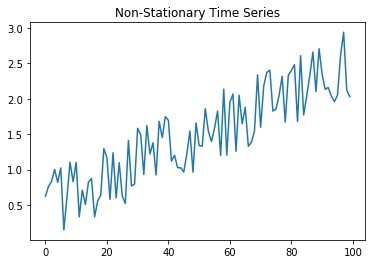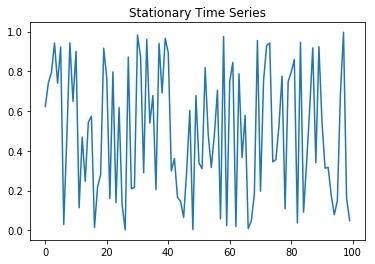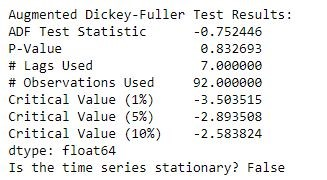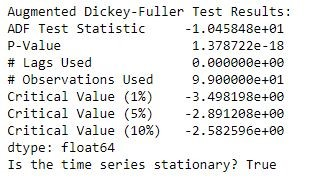Augmented Dickey-Fuller Test in Python

When performing time series analysis, most statistical forecasting methods assume that the time series is approximately stationary. How can you determine if a time series is stationary? The Augmented Dickey-Fuller Test is a well known statistical test that can help determine if your time series is stationary. In this article I will show you how to perform the Augmented Dickey-Fuller Test (ADF) test in python.

Stationary vs. Non-Stationary

In a stationary time series, statistical properties such as mean and variance are constant over time. In a non-stationary series, these properties are dependent on time.

Here is an example of a Non-Stationary time series:

import matplotlib.pyplot as plt
import random
random.seed(5)

non_stationary_series = []
for i in range(0,100):
non_stationary_series.append(random.random()+(i*.02))

plt.plot(non_stationary_series)
plt.title('Non-Stationary Time Series')
plt.show()There is clearly a trend in the above series of 100 randomly generated data points. Take a closer look at the code and you’ll easily spot the 2% being multiplied to the time component (i).

In a Stationary time series, there is no visible trend. Here is an example of a Stationary time series.

import matplotlib.pyplot as plt
import random
random.seed(5)

stationary_series = []
for i in range(0,100):
stationary_series.append(random.random())

plt.plot(stationary_series)
plt.title('Stationary Time Series')
plt.show()Augmented Dickey-Fuller Test (ADF) Statistical Test

To determine if a time series is stationary or not, we will use the ADF test which is a type of unit root test. Unit roots are a cause for non-stationarity, the ADF test will test if unit root is present.

A time series is stationary if a single shift in time doesn't change the time series statistical properties, in which case unit root does not exist.

The Null and Alternate hypothesis of the Augmented Dickey-Fuller test are defined as follows:

• Null Hypothesis states there is presence of a unit root.
• Alternate Hypothesis states there is no unit root. In other words, Stationarity exists.

To implement the ADF test in python, we will be using the statsmodel implementation. Statsmodels is a Python module that provides functions and classes for the estimation of many statistical models. The function to perform ADF is called adfuller.

First, import the required dependencies. Import the statsmodel module and the adfuller class from the tsa.statstools namespace. We will also need Pandas.

import statsmodels
from statsmodels.tsa.stattools import adfuller
import pandas as pd

We will create a class called StationarityTests to hold the ADF function. Our class constructor accepts a significance level as a parameter. This is defaulted to a significance level of 5%. It also contains an isStationary variable that will hold the results of the Augmented Dickey-Fuller test. If the time series is stationary, isStationary will be True, otherwise it will be False.

class StationarityTests:
def __init__(self, significance=.05):
self.SignificanceLevel = significance
self.pValue = None
self.isStationary = None

Lastly, we add the ADF implementation via a function called ADF_Stationarity_Test. This function takes a 1d-Array as input and a variable defaulted to True to determine if the function should print the full ADF results. The Akaike Information Criterion (AIC) is used to determine the lag.

The adfuller function returns a tuple of statistics from the ADF test such as the Test Statistic, P -Value, Number of Lags Used, Number of Observations used for the ADF regression and a dictionary of Critical Values.

If the P-Value is less than the Significance Level defined, we reject the Null Hypothesis that the time series contains a unit root. In other words, by rejecting the Null hypothesis, we can conclude that the time series is stationary.

If the pValue is very close to your significance level, you can use the Critical Values to help you reach a conclusion regarding the stationarity of your time series.

def ADF_Stationarity_Test(self, timeseries, printResults = True):

#Dickey-Fuller test:

if (self.pValue<self.SignificanceLevel):
self.isStationary = True
else:
self.isStationary = False

if printResults:
dfResults = pd.Series(adfTest[0:4], index=['ADF Test Statistic','P-Value','# Lags Used','# Observations Used'])

for key,value in adfTest.items():
dfResults['Critical Value (%s)'%key] = value

print('Augmented Dickey-Fuller Test Results:')
print(dfResults)

Testing Time Series for Stationarity

With our class now defined, its easy to test a time series for stationarity through the Augmented Dickey-Fuller test. Will test our first 2 series defined in this article for stationarity.

First, let’s see if our non_stationary_series is stationary. Instantiate the class and provide the non_stationary_series to the ADF_Stationarity_Test function like below. Then, print the results which will be holded by our isStationary variable in the class.

sTest = StationarityTests()
sTest.ADF_Stationarity_Test(non_stationary_series, printResults = True)
print("Is the time series stationary? {0}".format(sTest.isStationary))In this case, it is easy to see that the series is not stationary. P-Value of 0.83 is greater than our 5% significance level, therefore we fail to reject the null hypothesis that unit root does exist.

Another way to interpret this test is using the critical value which comes up to -0.75. This is greater than the 5% critical value of -2.89 (or the significance that you need) and therefore we fail to reject the null hypothesis.

Let’s run this same test but on our stationary series.

sTest = StationarityTests()
sTest.ADF_Stationarity_Test(stationary_series, printResults = True)
print("Is the time series stationary? {0}".format(sTest.isStationary))As you would expect, the results show that the series is actually stationary. In this case, the P-Value from our ADF test is much smaller than our 5% significance level, therefore we can reject the Null hypothesis and instead accept the alternate hypothesis that stationarity exists.

Taking a look at the critical value yields the same conclusion. The tests critical value ends up being -10.458 which is much smaller than the 5% critical value of -2.89 and so we have enough evidence to conclude that unit root does not exist. In other words, series is stationary.

Conclusion

You have now learned how to test for stationarity using the Augmented Dickey-Fuller Test (ADF) and are able to interpret the test using the P-Value or the Critical Values returned by the test. We created our own class which implements the ADF test from the statsmodels python package. Knowledge of this statistical test will greatly help you when you are building time series forecasting models in which stationarity is many times a strong underlying assumption for various models.